# Advent of Code F# – Day 25

Check out my new course Learn you some Lambda best practice for great good! and learn the best practices for performance, cost, security, resilience, observability and scalability.

The source code for this post is available here and you can click here to see my solutions for the other Advent of Code challenges.

Description for today’s challenge is here.

It’s the last day of the advent calendar, and today’s challenge appears difficult at first but mostly because of the grid system it imposes upon you.

But after some thought, the problem can be drastically simplified.

Given this grid system: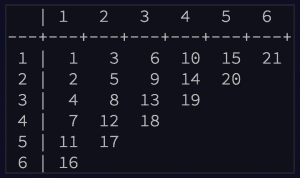we can work out the one-based index for row N, column 1 in this formula:

F N = F(N-1) + (N-1) where F 1 = 1, F 2 = 2

e.g.

F 2 = 2,

F 3 = F 2 + (3 – 1) = 2 + 2 = 4

F 4 = F 3 + (4 – 1) = 4 + 3 = 7

From here, column M is (M – 1) distance away from the first element on the same row and (M – 1) distance away from the row N that marks the start of the diagonal: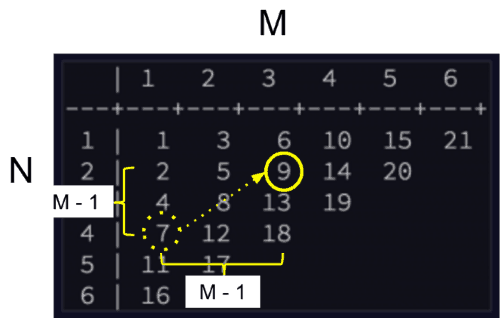So, the one-based index for row N, column M can be expressed in terms of the F function above:

G (M, N) = F (N + M – 1) + (M – 1)

e.g.

G (3, 2) = F (2 + 3 – 1) + (3 – 1) = F 4 + 2 = 7 + 2 = 9

G (6, 1) = F (1 + 6 – 1) + (6 – 1) = F 6 + 5 = 16 + 5 = 21

So for my puzzle input of row 2981, column 3075, it translates = G (3075, 2981)th element where the first element is 20151125.

Taking all these into account, and translating G (M, N) into an (x, y) based system, we can have the following to solve today’s challenge: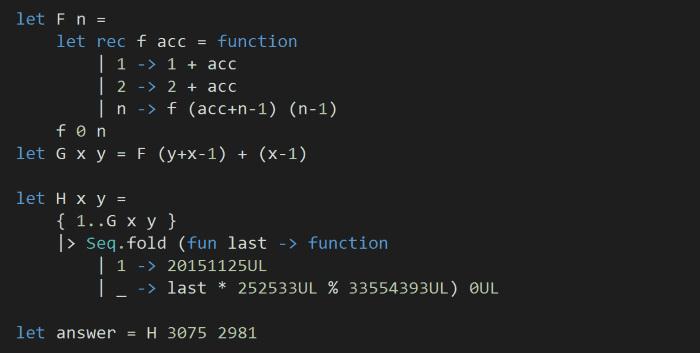Pretty straightforward, right? Well, the only caveat is how we implemented F here, this is only because I wanted the implementation to be tail recursive, otherwise it can be simple as:

let F = function | 1 -> 1 | 2 -> 2 | n -> F (n-1) + (n-1)

So that’s it folks! We’ve completed all the Advent of Code challenges for 2015, and our F# xmas tree is up and running in all its glory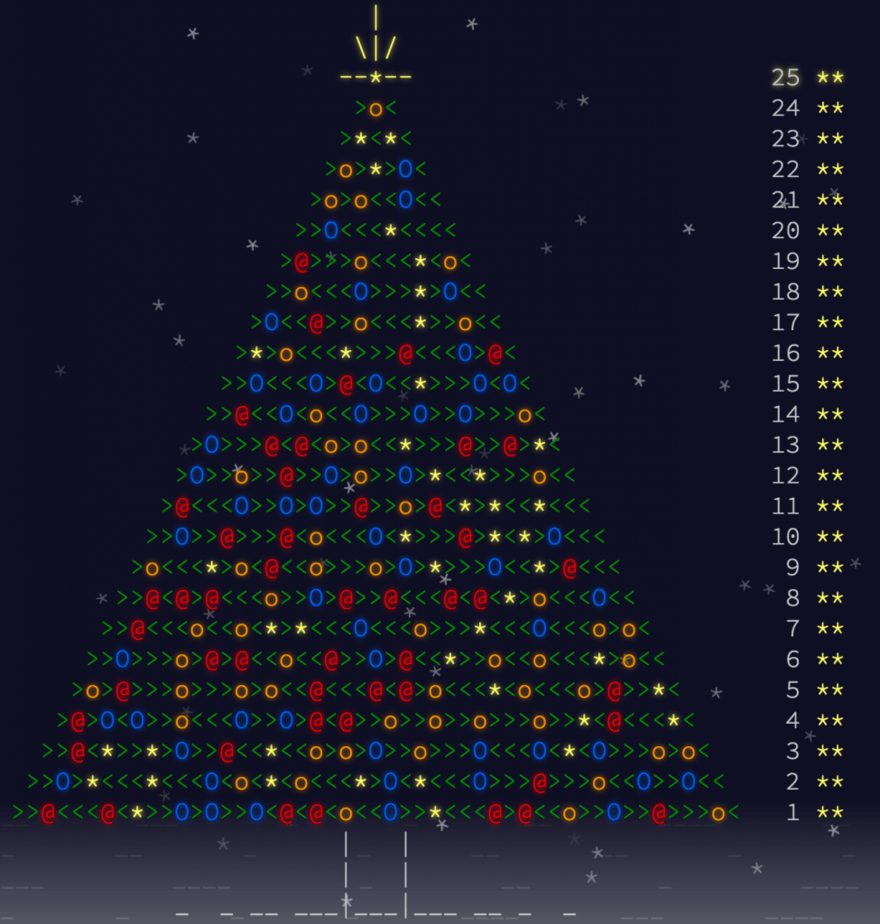Hi, I’m Yan. I’m an AWS Serverless Hero and the author of Production-Ready Serverless.

I specialise in rapidly transitioning teams to serverless and building production-ready services on AWS.

Are you struggling with serverless or need guidance on best practices? Do you want someone to review your architecture and help you avoid costly mistakes down the line? Whatever the case, I’m here to help.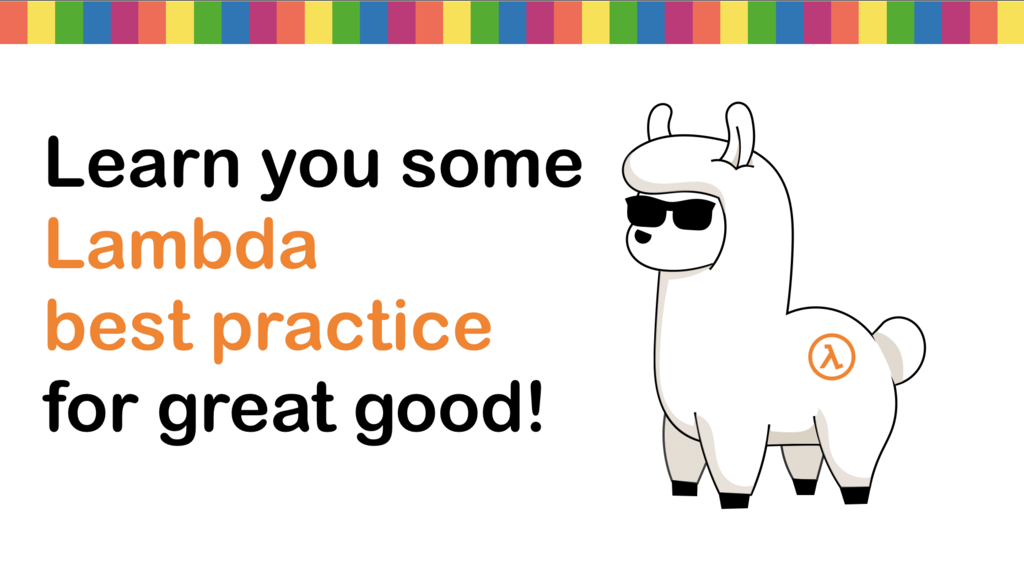Check out my new course, Learn you some Lambda best practice for great good! In this course, you will learn best practices for working with AWS Lambda in terms of performance, cost, security, scalability, resilience and observability. Enrol now and enjoy a special preorder price of £9.99 (~\$13).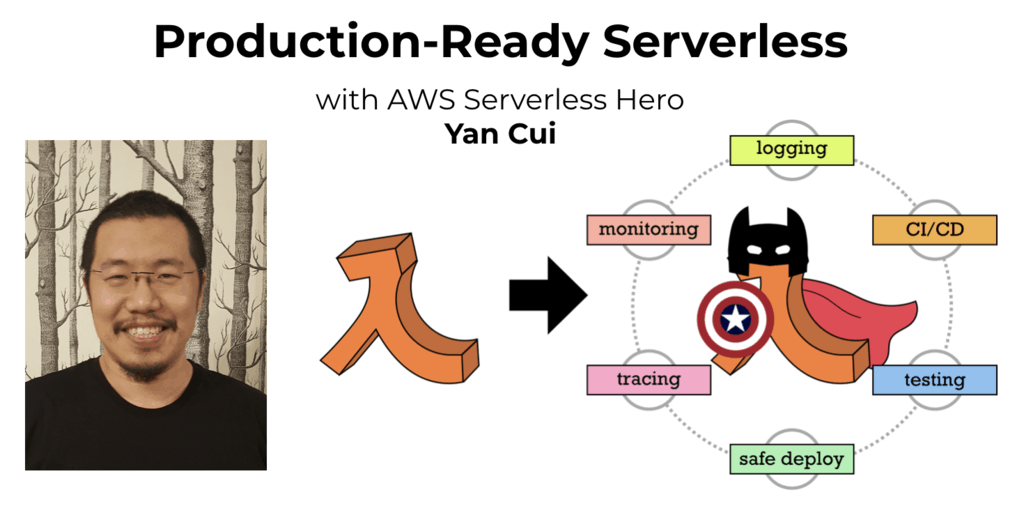Are you working with Serverless and looking for expert training to level-up your skills? Or are you looking for a solid foundation to start from? Look no further, register for my Production-Ready Serverless workshop to learn how to build production-grade Serverless applications!